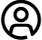# Calculators

Calculators are small handheld devices designed to make quick mathematical calculations. calculators are normally either battery or solar powered, but can also come two way powered (solar and battery powered)which ensures back up if batteries run out. Small calculators can also be known as pocket calculators or desktop calculators.

There are different types of calculators used for different functions:

Basic calculator This is the simplest and most popular calculator and offers functions like add, subtract, divide, multiply and percentages for basic mathematics.

Scientific calculators - These have more functions than the basic calculator includes functions like fractions, square root, degrees, combinations, trigonometric, logarithm and much more, used by students, engineers and scientists.

Graphical calculators - These are more advanced than the scientific calculators and incorporate a larger screen and bigger memory. It is able to convert data into graphs and plot data, they are also programmable. Widely used by students, engineers and scientists. Graphical calculators can normally be connected to another calculator or a pc to transfer data.

Printing calculators Provide instant printouts of the calculations these are used widely by accountants and in the financial sector. Normally larger than normal calculators, with larger keys and a bigger screen. The print out gives the user the ability to review their entered calculations and verify them.

27
Αποτελέσματα
Εφαρμοζόμενα Φίλτρα
Φίλτρα
Εμφάνιση:
Αποτελέσματα ανά σελίδα
20
• 20
• 50
• 100
 Τιμή Η αναγραφόμενη τιμή δεν περιλαμβάνει ΦΠΑ Λεπτομέρειες προϊόντος Brand Stock Type Display Lines Characters/Digits Per Line Power Source Battery Included Weight Battery Size Height Length Colour Width